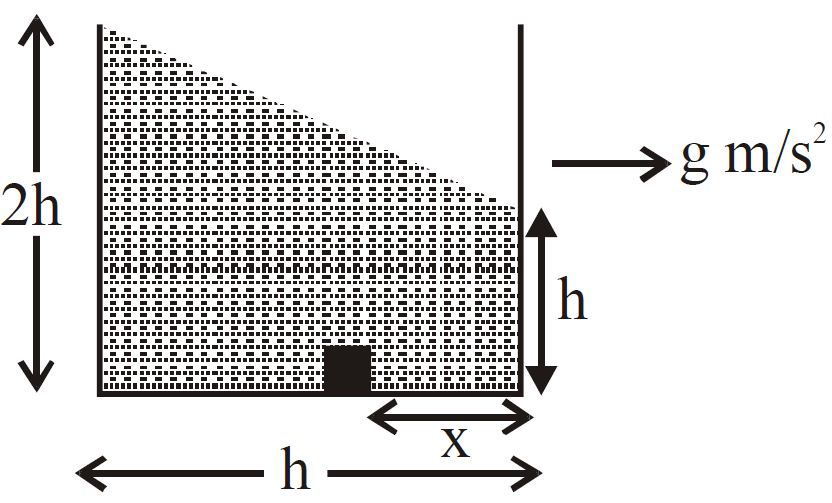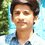# Question - 1.

A vessel is completely filled by a liquid of density $\rho$ as shown in figure having acceleration $g$ in horizontal direction. After getting equilibrium condition a particle of density $\frac{\rho}{2}$ is released from bottom of vessel at distance $x$ as shown in figure such that it reaches to water-air interface after time $\sqrt{\frac{2h}{g}}$. Assume all collisions with wall are completely elastic. Which of the positions satisfy the given situation

$A)$ $x=0$

$B )$ $x=h$

$C)$ $x=\frac{h}{9}$

$D)$ $x=\frac{h}{4}$Note by Nishant Rai
6 years, 2 months ago

This discussion board is a place to discuss our Daily Challenges and the math and science related to those challenges. Explanations are more than just a solution — they should explain the steps and thinking strategies that you used to obtain the solution. Comments should further the discussion of math and science.

When posting on Brilliant:

• Use the emojis to react to an explanation, whether you're congratulating a job well done , or just really confused .
• Ask specific questions about the challenge or the steps in somebody's explanation. Well-posed questions can add a lot to the discussion, but posting "I don't understand!" doesn't help anyone.
• Try to contribute something new to the discussion, whether it is an extension, generalization or other idea related to the challenge.

MarkdownAppears as
*italics* or _italics_ italics
**bold** or __bold__ bold
- bulleted- list
• bulleted
• list
1. numbered2. list
1. numbered
2. list
Note: you must add a full line of space before and after lists for them to show up correctly
paragraph 1paragraph 2

paragraph 1

paragraph 2

[example link](https://brilliant.org)example link
> This is a quote
This is a quote
    # I indented these lines
# 4 spaces, and now they show
# up as a code block.

print "hello world"
# I indented these lines
# 4 spaces, and now they show
# up as a code block.

print "hello world"
MathAppears as
Remember to wrap math in $$ ... $$ or $ ... $ to ensure proper formatting.
2 \times 3 $2 \times 3$
2^{34} $2^{34}$
a_{i-1} $a_{i-1}$
\frac{2}{3} $\frac{2}{3}$
\sqrt{2} $\sqrt{2}$
\sum_{i=1}^3 $\sum_{i=1}^3$
\sin \theta $\sin \theta$
\boxed{123} $\boxed{123}$

Sort by:

- 6 years, 2 months ago

- 6 years, 2 months ago

- 6 years, 2 months ago

Oh yes, I have seen this problem. This is a multiple correct problem. The answer is a,b,c if I remember right.

- 6 years, 2 months ago

Correct! This question came in my mock test, and i am still unable to solve it.

- 6 years, 2 months ago

Getting options a and b is relatively easy. You just need to use the concept of effective acceleration due to gravity.

- 6 years, 2 months ago

can you please write the solution. (i tried, but in vain )

- 6 years, 2 months ago

I have not gotten it either. One of my friends showed me this problem and I failed to get answer. I tried now and I still havent gotten it. :/

- 6 years, 2 months ago